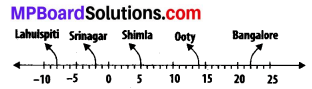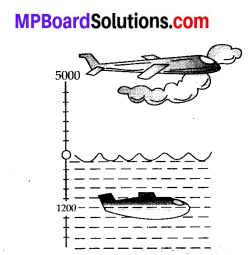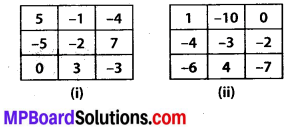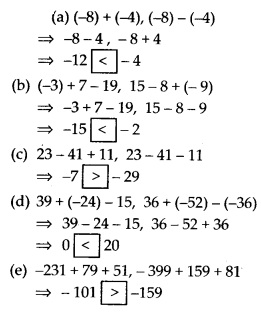# MP Board Class 7th Maths Solutions Chapter 1 Integers Ex 1.1

In this article, we will share MP Board Class 7th Maths Solutions Chapter 1 Integers Ex 1.1 Pdf, These solutions are solved subject experts from the latest edition books.

## MP Board Class 7th Maths Solutions Chapter 1 Integers Ex 1.1

Question 1.
Following number line shows the temperature in degree Celsius (°C) at different places on a particular day.(a) Observe this number line and write the temperature of the places marked on it.
(b) What is the temperature difference between the hottest and the coldest places among the above?
(c) What is the temperature difference between Lahulspiti and Srinagar?
(d) Can we say temperature of Srinagar and Shimla taken together is less than the temperature at Shimla? Is it also less than the temperature at Srinagar?
Solution:
(a) The temperature of the cities are as follows:
Lahulspiti: -8°C; Srinagar : – 2°C; Shimla : 5°C; Ooty : 14°C; Bangalore : 22°C

(b) Temperature at the hottest place,
i. e., Bangalore = 22°C
Temperature at the coldest place,
i. e., Lahulspiti = – 8°C.
∴ Temperature difference = 22° – (-8°C)
= 30°C

(c) Temperature at Lahulspiti = – 8°C;
Temperature at Srinagar = – 2°C
∴ Temperature difference = – 2°C – (-8°C)
= 6° C

(d) Temperature at Srinagar = -2°C;
Temperature at Shimla = 5°C
Temperature of Srinagar and Shimla taken together = – 2°C + 5°C = 3°C
Hence, the temperature of Srinagar and Shimla taken together is less than the temperature at Shimla but greater than the temperature at Srinagar.Question 2.
In a quiz, positive marks are given for correct answers and negative marks are given for incorrect answers. If Jack’s scores in five successive rounds were 25, – 5, – 10, 15 and
Solution:
Total score of Jack at the end will be the sum of scores in five successive rounds.
∴ Jack’s total score at the end
= 25 – 5 – 10 + 15 + 10 = 35

Question 3.
At Srinagar temperature was – 5°C on Monday and then it dropped by 2°C on Tuesday. What was the temperature of Srinagar on Tuesday? On Wednesday, it rose by 4°C. What was the temperature on this day?
Solution:
Temperature on Monday = – 5°C
Temperature on Tuesday = Temperature on Monday – 2°C = -5°C – 2°C = – 7°C Temperature on Wednesday = Temperature on Tuesday + 4°C = – 7°C + 4°C = – 3°CQuestion 4.
A plane is flying at the height of 5000 m above the sea level. At a particular point, it is exactly above a submarine floating 1200 m below the sea level. What is the vertical distance between them?Height of plane = 5000 m
Depth of submarine = -1200 m
Distance between plane and submarine = 5000 – (-1200) = 5000 + 1200 = 6200 m

Question 5.
Mohan deposits ₹ 2,000 in his bank account and withdraws ₹ 1,642 from it, the next day. If withdrawal of amount from the account is represented by a negative integer, then how will you represent the amount deposited? Find the balance in Mohan’s account after the withdrawal.
Solution:
Since the amount withdrawn is represented by a negative integer, the amount deposited will be represented by a positive integer.
Amount deposited = ₹ 2000
Amount withdrawn = – ₹ 1642 Balance in Mohan’s account = Money deposited + Money withdrawn = 2000 + (-1642) = 2000 – 1642 = ₹ 358Question 6.
Rita goes 20 km towards east from a point A to the point B. From B, she moves 30 km towards west along the same road. If the distance towards east is represented by a positive integer then, how will you represent the distance travelled towards west? By which integer will you represent her final position from A?Solution:
Since, the distance towards east is represented by a positive integer, then the distance travelled towards west will be represented by a negative integer.
Distance travelled in east direction = 20 km
Distance travelled in west direction = – 30 km
Distance travelled from A = 20 + (-30)
= -10 km
Therefore, we will represent the distance travelled by Rita from point A by a negative integer, i.e., -10 km (i.e., Rita is now in west direction).

Question 7.
In a magic square each row, column and diagonal have the same sum. Check which of the following is a magic square.Solution:
It can be observed that in square (i), every row and column add up to give 0. However, the sum of one of its diagonals is not 0.
As -4 – 2 = -6 ≠ 0,
Therefore,
(i) is not a magic square. Similarly, in square
(ii), each row, column, and diagonal add up to give – 9. Therefore,
(iii) is a magic square.Question 8.
Verify a – (- b) = a + b for the following values of a and b.
(i) a = 21, b = 18
(ii) a = 118,b = 125
(iii) a = 75, b = 84
(iv) a = 28, b = 11
Solution:
(i) a = 21, b = 18; a + b = 21 + 18 = 39
a – (- b) = 21 – (-18) = 21 + 18 = 39

(ii) a = 118, b = 125;
a – (-b) = 118 – (-125) = 118 + 125 = 243
a + b = 118 + 125 = 243

(iii) a = 75,b = 84;
a – (-b) = 75- (-84) = 75 + 84 = 159
a + b = 75 + 84 = 159

(iv) a = 28, b = 11;
a – (-b) = 28 – (-11) = 28 + 11 = 39
a + b = 28 + 11 = 39

Question 9.
Use the sign of >, < or = in the box to make the statements true.Solution:Question 10.
A water tank has steps inside it. A monkey is sitting on the topmost step (i.e., the first step). The water level is at the ninth step.
(i) He jumps 3 steps down and then jumps back 2 steps up. In how many jumps will he reach the water level?
(ii) After drinking water, he wants to go back. For this, he jumps 4 steps up and then jumps back 2 steps down in every move. In how many jumps will he reach back the top step?
(iii) If the number of steps moved down is represented by negative integers and the number of steps moved up by positive integers, represent his moves in part (i) and (ii) by completing the following;
(a) – 3 + 2 -… = – 8
(b) 4 – 2 + … = 8.
In (a) the sum (- 8) represents going down by eight steps. So, what will the sum 8 in (b) represent?
Solution:
Let the number of steps moved down be represented by positive integers and the number of steps moved up be represented by negative integers.
(i) Initially, the monkey was at the 1st step. The monkey will be at step:
After 1st jump =1 + 3 = 4
After 2nd jump = 4 + (-2) = 2
After 3rd jump = 2 + 3 = 5
After 4th jump = 5 + (-2) = 3
After 5th jump = 3 + 3 = 6
After 6th jump = 6 + (-2) = 4
After 7th jump = 4 + 3 = 7
After 8th jump = 7 + (-2) = 5
After 9th jump = 5 + 3 = 8
After 10th jump = 8 + (-2) = 6
After 11th jump = 6 + 3 = 9
Hence, the monkey will reach the water level after 11 jumps.

(ii) Initially, the monkey was at step = 9 The monkey will be at step:
After 1st jump = 9 + (- 4) = 5
After 2nd jump = 5 + 2 = 7
After 3rd jump = 7 + (- 4) = 3
After 4th jump = 3 + 2 = 5
After 5th jump = 5 + (- 4) = 1
Clearly, the monkey will reach back the top step after 5 jumps.

(iii) If number of steps moved down is represented by negative integers and number of steps moved up is represented by positive integers, then his moves will be as follows:

Moves in part (i)

(a) -3 + 2 – 3 + 2 – 3 + 2 – 3 + 2 – 3 + 2 – 3
= -8
Moves in part (ii)

(b) 4 – 2 + 4 – 2 + 4 = 8
Moves in (b) represents going up 8 steps.